# Layers

## layer_id

Each layer requires a layer_id value. If you don’t supply one, it will create it for you, but this may have some unintended consequences for you.

The layer_id is the ID that deck.gl uses to ‘shallow-compare’ layers to see if they need updating. Which comes in handy when working in Shiny. If you update data, for example when using a reactive() function, and use the same layer_id, deck.gl knows it should update the layer rather than re-draw it completely.

However, if two of your layers of the same type have the same layer_id, then deck.gl will get confused and will only show the last layer drawn.

For example, if you have to scatterplot layers, both with the same layer_id, only the second one will be shown.

## Arcs

url <- 'https://raw.githubusercontent.com/plotly/datasets/master/2011_february_aa_flight_paths.csv'
flights$id <- seq_len(nrow(flights)) flights$stroke <- sample(1:3, size = nrow(flights), replace = T)

mapdeck( token = key, style = mapdeck_style("dark"), pitch = 45 ) %>%
data = flights
, layer_id = "arc_layer"
, origin = c("start_lon", "start_lat")
, destination = c("end_lon", "end_lat")
, stroke_from = "airport1"
, stroke_to = "airport2"
, stroke_width = "stroke"
)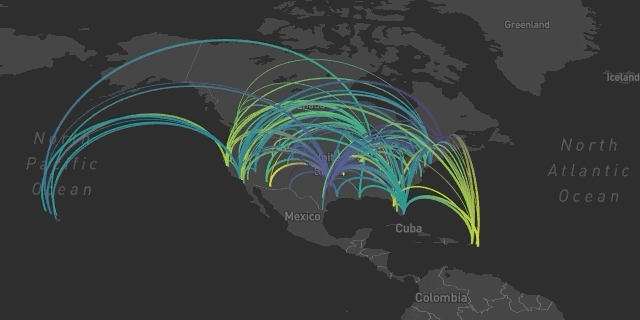Arcs

## Animated Arcs

mapdeck( token = key, style = 'mapbox://styles/mapbox/dark-v9', pitch = 45 ) %>%
data = flights
, layer_id = "arc_layer"
, origin = c("start_lon", "start_lat")
, destination = c("end_lon", "end_lat")
, stroke_from = "airport1"
, stroke_to = "airport2"
, stroke_width = "stroke"
)

Arcs

## GeoJSON

mapdeck(
token = key
, pitch = 35
) %>%
data = geojson
, layer_id = "geojson"
)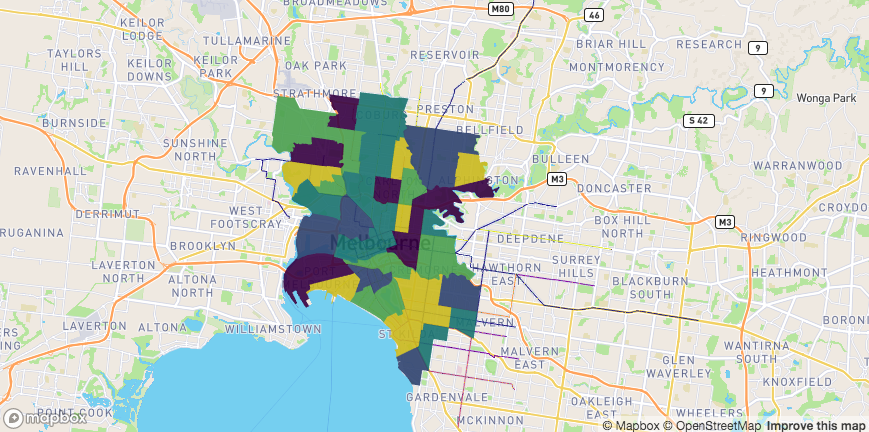GeoJSON

## Great Circles

url <- 'https://raw.githubusercontent.com/plotly/datasets/master/2011_february_aa_flight_paths.csv'
flights$id <- seq_len(nrow(flights)) flights$stroke <- sample(1:3, size = nrow(flights), replace = T)

mapdeck( style = 'mapbox://styles/mapbox/dark-v9') %>%
data = flights
, layer_id = "arc_layer"
, origin = c("start_lon", "start_lat")
, destination = c("end_lon", "end_lat")
, stroke_from = "airport1"
, stroke_to = "airport2"
, stroke_width = "stroke"
)Great Circles

## Grid

df <- read.csv(paste0(
'https://raw.githubusercontent.com/uber-common/deck.gl-data/master/',
'examples/3d-heatmap/heatmap-data.csv'
))

df <- df[!is.na(df$lng), ] mapdeck( token = key, style = mapdeck_style("dark"), pitch = 45 ) %>% add_grid( data = df , lat = "lat" , lon = "lng" , cell_size = 5000 , elevation_scale = 50 , layer_id = "grid_layer" )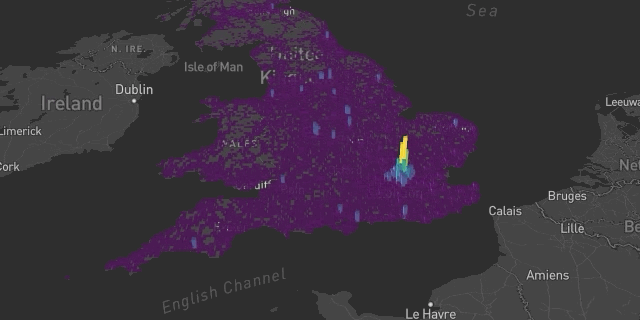Grid ## Heatmap df <- read.csv(paste0( 'https://raw.githubusercontent.com/uber-common/deck.gl-data/master/', 'examples/3d-heatmap/heatmap-data.csv' )) df <- df[!is.na(df$lng), ]

mapdeck( style = mapdeck_style('dark'), pitch = 45 ) %>%
data = df[1:30000, ]
, lat = "lat"
, lon = "lng"
, weight = "weight",
, colour_range = colourvalues::colour_values(1:6, palette = "inferno")
)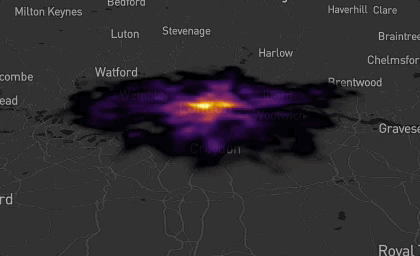Heatmap

## Hexagons


library(mapdeck)

'https://raw.githubusercontent.com/uber-common/deck.gl-data/master/examples/'
, '3d-heatmap/heatmap-data.csv'
))

df <- df[!is.na(df$lng), ] mapdeck( style = mapdeck_style("dark"), pitch = 45) %>% add_hexagon( data = df[ df$lat > 54.5, ]
, lat = "lat"
, lon = "lng"
, layer_id = "hex_layer"
, elevation_scale = 100
, colour_range = colourvalues::colour_values(1:6, palette = colourvalues::get_palette("viridis")[70:256,])
)Hexagons

## Lines

url <- 'https://raw.githubusercontent.com/plotly/datasets/master/2011_february_aa_flight_paths.csv'
flights$id <- seq_len(nrow(flights)) flights$stroke <- sample(1:3, size = nrow(flights), replace = T)

mapdeck( token = key, style = mapdeck_style("dark"), pitch = 45 ) %>%
data = flights
, layer_id = "line_layer"
, origin = c("start_lon", "start_lat")
, destination = c("end_lon", "end_lat")
, stroke_colour = "airport1"
, stroke_width = "stroke"
)Lines

## Path

mapdeck(
token = key
, style = mapdeck_style("dark")
, zoom = 10) %>%
, stroke_colour = "RIGHT_LOC"
, layer_id = "path_layer"
)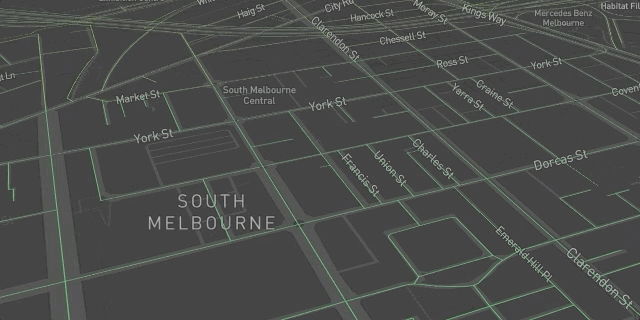Path

## Point cloud

df <- capitals
df$z <- sample(10000:10000000, size = nrow(df)) mapdeck(token = key, style = mapdeck_style("dark")) %>% add_pointcloud( data = df , lon = 'lon' , lat = 'lat' , elevation = 'z' , layer_id = 'point' , fill_colour = "country" )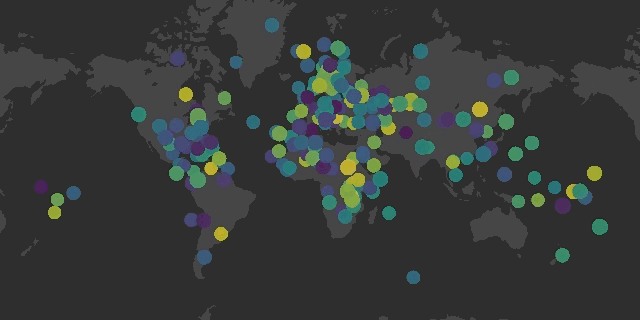Pointcloud ## Polygons library(sf) library(geojsonsf) sf <- geojson_sf("https://symbolixau.github.io/data/geojson/SA2_2016_VIC.json") sf$e <- sf$AREASQKM16 * 10 mapdeck(token = key, style = mapdeck_style("dark")) %>% add_polygon( data = sf[ data.table::%like%(sf$SA4_NAME16, "Melbourne"), ]
, layer = "polygon_layer"
, fill_colour = "SA2_NAME16"
, elevation = "e"
)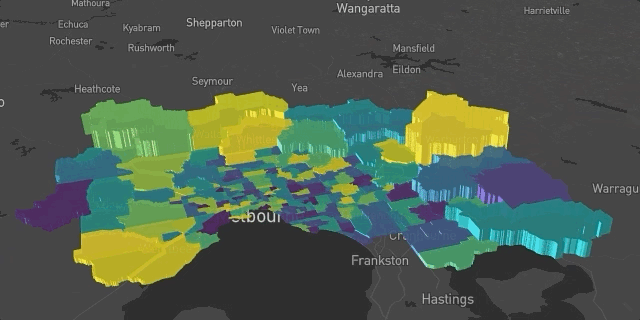Polygons

## Scatter

mapdeck( token = key ) %>%
data = capitals
, lat = "lat"
, lon = "lon"
, fill_colour = "country"
, layer_id = "scatter_layer"
, palette = "plasma"
)Scatter

## Screen grid

df <- read.csv(paste0(
'https://raw.githubusercontent.com/uber-common/deck.gl-data/master/',
'examples/3d-heatmap/heatmap-data.csv'
))

df <- df[ !is.na(df$lng), ] df$weight <- sample(1:10, size = nrow(df), replace = T)

mapdeck( token = key, style = mapdeck_style('dark'), pitch = 45 ) %>%
data = df
, lat = "lat"
, lon = "lng"
, weight = "weight"
, layer_id = "screengrid_layer"
, cell_size = 10
, opacity = 0.3
, colour_range = colourvalues::colour_values(1:6, palette = "plasma")
)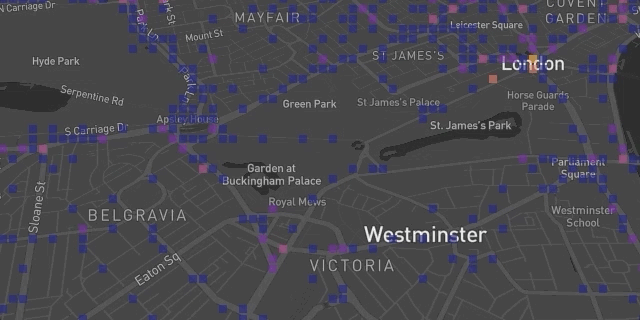Screengrid

## Text

mapdeck(token = key, style = mapdeck_style('dark')) %>%
data = capitals
, lon = 'lon'
, lat = 'lat'
, fill_colour = 'country'
, text = 'capital'
, layer_id = 'text'
, size = 16
)Text

## Multiple layers

df1 <- capitals[ capitals$country == "Australia", ] df2 <- capitals[ capitals$country != "Australia", ]
df1$key <- 1L df2$key <- 1L

df <- merge(df1, df2, by = 'key')

mapdeck(
token = key
, style = mapdeck_style("dark")
, pitch = 35
) %>%
data = df
, origin = c("lon.x", "lat.x")
, destination = c("lon.y", "lat.y")
, layer_id = "arc_layer"
, stroke_from = "country.x"
, stroke_to = "country.y"
, stroke_width = 2
) %>%
data = df2
, lon = "lon"
, lat = "lat"
, fill_colour = "country"
, layer_id = "scatter"
)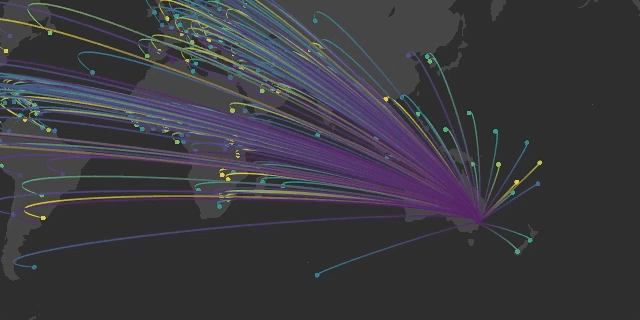Multiple Layers

# Shiny

The three main functions to use are

1. renderMapdeck() in the Server
2. mapdeckOutput() in the UI
3. mapdeck_update() to update an existing map

You can observe interactions with the layers by using observeEvent(). The event you observe is formed by combining

'map_id + "_" + layer + "_click"

where

• map_id is the outputId of the mapdeckOutput() function
• layer is the layer you’re interacting with
• _click is you observing ‘clicking’ on the layer

There’s an example of this in the shiny.

library(shiny)
library(shinydashboard)
library(jsonify)

ui <- dashboardPage(
, dashboardSidebar()
, dashboardBody(
mapdeckOutput(
outputId = 'myMap'
),
sliderInput(
inputId = "longitudes"
, label = "Longitudes"
, min = -180
, max = 180
, value = c(-180,-90)
)
, verbatimTextOutput(
outputId = "observed_click"
)
)
)
server <- function(input, output) {

origin <- capitals[capitals$country == "Australia", ] destination <- capitals[capitals$country != "Australia", ]
origin$key <- 1L destination$key <- 1L

df <- merge(origin, destination, by = 'key', all = T)

output$myMap <- renderMapdeck({ mapdeck(style = mapdeck_style('dark')) }) ## plot points & lines according to the selected longitudes df_reactive <- reactive({ if(is.null(input$longitudes)) return(NULL)
lons <- input$longitudes return( df[df$lon.y >= lons & df$lon.y <= lons, ] ) }) observeEvent({input$longitudes}, {
if(is.null(input$longitudes)) return() mapdeck_update(map_id = 'myMap') %>% add_scatterplot( data = df_reactive() , lon = "lon.y" , lat = "lat.y" , fill_colour = "country.y" , radius = 100000 , layer_id = "myScatterLayer" , update_view = FALSE ) %>% add_arc( data = df_reactive() , origin = c("lon.x", "lat.x") , destination = c("lon.y", "lat.y") , stroke_from = "country.x" , stroke_to = "country.y" , layer_id = "myArcLayer" , id = "country.x" , stroke_width = 4 , update_view = FALSE ) }) ## observe clicking on a line and return the text observeEvent(input$myMap_arc_click, {

event <- input$myMap_arc_click output$observed_click <- renderText({
jsonify::pretty_json( event )
})
})
}

shinyApp(ui, server)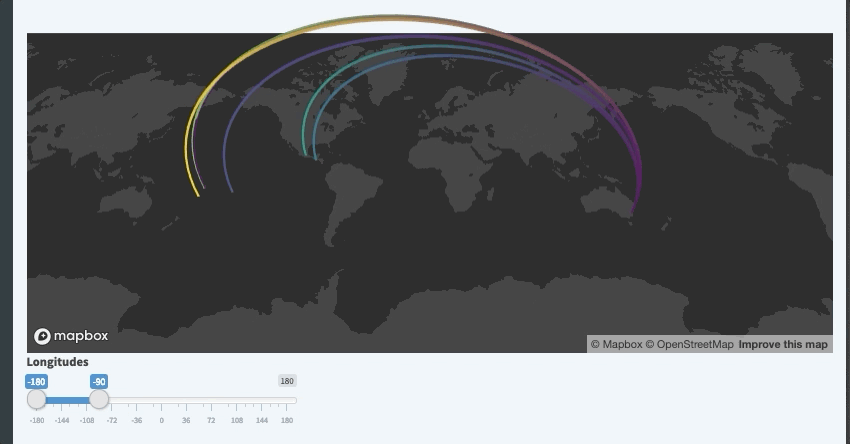Shiny